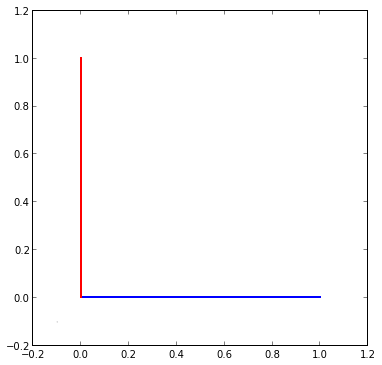# How To Draw Lines With Matplotlib

Simple example to show how to draw lines with Matplotlib (in IPython Notebook).

ipython notebook --pylab inline


In :
import matplotlib.lines as lines
fig, ax = plt.subplots()

fig.set_size_inches(6,6)          # Make graph square
scatter([-0.1],[-0.1],s=0.01)     # Move graph window a little left and down

line1 = [(0,0), (1,0)]
line2 = [(0,0), (0,1)]

# Note that the Line2D takes a list of x values and a list of y values,
# not 2 points as one might expect.  So we have to convert our points
# an x-list and a y-list.
(line1_xs, line1_ys) = zip(*line1)
(line2_xs, line2_ys) = zip(*line2)